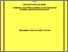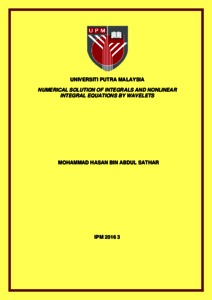# Numerical solution of integrals and nonlinear integral equations by wavelets

## Citation

Abdul Sathar, Mohammad Hasan (2016) Numerical solution of integrals and nonlinear integral equations by wavelets. Doctoral thesis, Universiti Putra Malaysia.

## Abstract

In recent years, wavelets have found their way into many different fields of science and engineering. This is because wavelets possess several important properties, such as orthogonality, compact support, exact representation of polynomials at certain degree and the ability to represent functions on different levels of resolution. In this thesis, new methods based on wavelet expansion are considered to solve problems arising in approximation of functions, integrals and integral equations. Mainly we deal with the numerical approximations by Haar wavelets, linear Legendre multiwavelets and Chebyshev wavelets. Numerous work has been done to solve numerical integration in terms of quadrature rule. Regardless of the simplicity of quadrature rule, there exist some drawbacks. In order to overcome these existing drawbacks, new methods based on Haar wavelets and linear Legendre multi-wavelets are proposed to obtain numerical solutions of double, triple and N dimensional integrals. Main advantages of these methods are its efficiency and simple applicability. Furthermore, the error analysis for double and triple integral where functions belong in the class of C2(R) and C3(R) is worked out to show the efficiency of the methods. The second part of the thesis focus on obtaining error estimations for the approximation by Haar and Chebyshev wavelets and linear Legendre multi wavelets. Error estimations are established for functions from Holder Hs[0;1] and Holder Zygmund Cm:a[0;1] classes. Therefore functions can be consider in a wider class compared to the previous work. The smoothness of functions from Holder and Holder Zygmund classes is reflected in the error estimation. Finally, new numerical techniques to solve nonlinear Fredholm and Volterra integral equation of the second kind by Haar and Chebyshev wavelets are developed. These methods reduce the nonlinear integral equation to a linear algebraic system of equation. Newton Kantorovich method is implemented to reduce the nonlinear integral equations into linear integral equations. This allows us to establish approximation solutions for nonlinear integrals. The comparison of error and accuracy between other methods are shown.Preview
Text
IPM 2016 3 - IR.pdfView Item